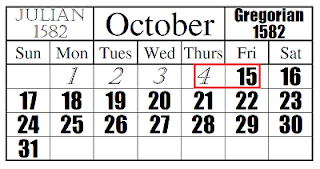# Why September, 1752 has only 19 days?

Your forefather slept on 2nd september, 1752 and woke the next day on 14th, september, 1752 missing altogether 11 days.  Why? Read on!!

The Julian calendar considered one year is equal to 365.25 days and started adding 1 day for every 4 years.  So every 4th year became leap year.  For our convenience, we started considering the years which are divisible by 4 leap years.

But later it is found that one solar year is equal to 365.24217898.  The difference is not really high but over a period of several centuries, this difference indeed started causing some sort of trouble.

To calculate Easter celebration dates, Pope Gregory XIII (1502 - 1585) faced some problem with the existing calendar system.  The existing calendar years are slightly longer so we are celebrating some important dates later than actual date.

For example, 365.25 - 365.24217898 = 0.00782101999 is very small difference but over a period of 1500 years, this difference became 1500 x 0.00782101999 = 11.73 days. This means, we tend to celebrate an important date like Christmas  around 11 days later than actual date.

To correct this anomaly, Pope Gregory called upon an Italian mathematician and scientist Alosius Lilius and asked him to make a new calendar which addresses the above issue.

Here again continued fractions come to our rescue.
$$0.24219878 = \dfrac{{24219878}}{{100000000}}$$$$0.24217898 = 0 + \cfrac{1}{{4 + \cfrac{1}{{7 + \cfrac{1}{{1 + \cfrac{1}{{3 + \cfrac{1}{{5 + ...}}}}}}}}}}$$

So the convergents are $$\dfrac{{{p_1}}}{{{q_1}}} = \dfrac{1}{4}$$, $$\dfrac{{{p_2}}}{{{q_2}}} = \dfrac{7}{{29}}$$, $$\dfrac{{{p_3}}}{{{q_3}}} = \dfrac{8}{{33}}$$, $$\dfrac{{{p_4}}}{{{q_4}}} = \dfrac{{31}}{{128}}$$, $$\dfrac{{{p_5}}}{{{q_5}}} = \dfrac{{163}}{{673}}$$

You can see that the first convergent = $$\dfrac{1}{4}$$ which we adopted for many years to calculate leap years.  If you look at other convergents they are difficult to remember and confusing.  For example, we can have 7 leap days for 29 years or 8 leap days for 33 years etc.  As the denominators of the above convergents don't facilitate easy calculations we have to consider much longer cycle of 100 year stretch.  So we multiply our fraction by 100 and later divide the convergent with 100.

$$0.219878 = \dfrac{{219878}}{{1000000}}$$$$0.24219878 \times 100 = 24 + \cfrac{1}{{4 + \cfrac{1}{{1 + \cfrac{1}{{1 + \cfrac{1}{{4 + ..}}}}}}}}$$

The convergents are  $$\dfrac{{{p_1}}}{{{q_1}}} = \dfrac{{97}}{4}$$, $$\dfrac{{{p_2}}}{{{q_2}}} = \dfrac{{121}}{{5}}$$, $$\dfrac{{{p_3}}}{{{q_3}}} = \dfrac{{218}}{9}$$, $$\dfrac{{{p_4}}}{{{q_4}}} = \dfrac{{993}}{{41}}$$

Now we can have 97 leap days for 400 years or 121 leap days for 500 years etc.  As we know that each subsequent convergent is a better approximation of the given decimal number, 121 leap days for 500 is better but why Pope Gregory has chosen 97 leap days for 400 is not known.  Also 218 leap days for 900 year cycle is very long and not practical.

So In 1582, Pope Gregory issued a papal bull ordering that 10 days to be removed from October 1582.  So October 1582 contained only 21 days that year.   But not all the countries adopted this new calendar especially Protestants.  They actually thought this is a conspiracy by catholic church.  Only Catholic churches and some orthodox churches adopted this new calendar.It took almost 200 years the Gregorian calendar became popular across the world.  As Britain is protestant country, Britain and the colonies including America changed their calendars in 1752 by removing 11 days from September 1752.